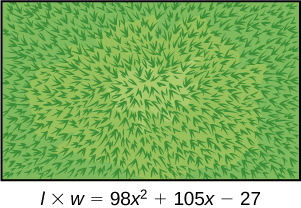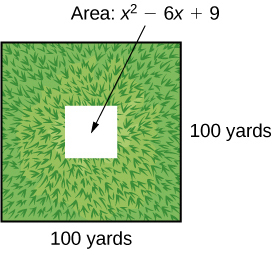# 1.5 Factoring polynomials  (Page 4/6)

 Page 4 / 6

Given a sum of cubes or difference of cubes, factor it.

1. Confirm that the first and last term are cubes, $\text{\hspace{0.17em}}{a}^{3}+{b}^{3}\text{\hspace{0.17em}}$ or $\text{\hspace{0.17em}}{a}^{3}-{b}^{3}.$
2. For a sum of cubes, write the factored form as $\text{\hspace{0.17em}}\left(a+b\right)\left({a}^{2}-ab+{b}^{2}\right).\text{\hspace{0.17em}}$ For a difference of cubes, write the factored form as $\text{\hspace{0.17em}}\left(a-b\right)\left({a}^{2}+ab+{b}^{2}\right).$

## Factoring a sum of cubes

Factor $\text{\hspace{0.17em}}{x}^{3}+512.$

Notice that $\text{\hspace{0.17em}}{x}^{3}\text{\hspace{0.17em}}$ and $\text{\hspace{0.17em}}512\text{\hspace{0.17em}}$ are cubes because $\text{\hspace{0.17em}}{8}^{3}=512.\text{\hspace{0.17em}}$ Rewrite the sum of cubes as $\text{\hspace{0.17em}}\left(x+8\right)\left({x}^{2}-8x+64\right).$

Factor the sum of cubes: $\text{\hspace{0.17em}}216{a}^{3}+{b}^{3}.$

$\left(6a+b\right)\left(36{a}^{2}-6ab+{b}^{2}\right)$

## Factoring a difference of cubes

Factor $\text{\hspace{0.17em}}8{x}^{3}-125.$

Notice that $\text{\hspace{0.17em}}8{x}^{3}\text{\hspace{0.17em}}$ and $\text{\hspace{0.17em}}125\text{\hspace{0.17em}}$ are cubes because $\text{\hspace{0.17em}}8{x}^{3}={\left(2x\right)}^{3}\text{\hspace{0.17em}}$ and $\text{\hspace{0.17em}}125={5}^{3}.\text{\hspace{0.17em}}$ Write the difference of cubes as $\text{\hspace{0.17em}}\left(2x-5\right)\left(4{x}^{2}+10x+25\right).$

Factor the difference of cubes: $\text{\hspace{0.17em}}1,000{x}^{3}-1.$

$\left(10x-1\right)\left(100{x}^{2}+10x+1\right)$

## Factoring expressions with fractional or negative exponents

Expressions with fractional or negative exponents can be factored by pulling out a GCF. Look for the variable or exponent that is common to each term of the expression and pull out that variable or exponent raised to the lowest power. These expressions follow the same factoring rules as those with integer exponents. For instance, $\text{\hspace{0.17em}}2{x}^{\frac{1}{4}}+5{x}^{\frac{3}{4}}\text{\hspace{0.17em}}$ can be factored by pulling out $\text{\hspace{0.17em}}{x}^{\frac{1}{4}}\text{\hspace{0.17em}}$ and being rewritten as $\text{\hspace{0.17em}}{x}^{\frac{1}{4}}\left(2+5{x}^{\frac{1}{2}}\right).$

## Factoring an expression with fractional or negative exponents

Factor $\text{\hspace{0.17em}}3x{\left(x+2\right)}^{\frac{-1}{3}}+4{\left(x+2\right)}^{\frac{2}{3}}.$

Factor out the term with the lowest value of the exponent. In this case, that would be $\text{\hspace{0.17em}}{\left(x+2\right)}^{-\frac{1}{3}}.$

Factor $\text{\hspace{0.17em}}2{\left(5a-1\right)}^{\frac{3}{4}}+7a{\left(5a-1\right)}^{-\frac{1}{4}}.$

${\left(5a-1\right)}^{-\frac{1}{4}}\left(17a-2\right)$

Access these online resources for additional instruction and practice with factoring polynomials.

## Key equations

 difference of squares ${a}^{2}-{b}^{2}=\left(a+b\right)\left(a-b\right)$ perfect square trinomial ${a}^{2}+2ab+{b}^{2}={\left(a+b\right)}^{2}$ sum of cubes ${a}^{3}+{b}^{3}=\left(a+b\right)\left({a}^{2}-ab+{b}^{2}\right)$ difference of cubes ${a}^{3}-{b}^{3}=\left(a-b\right)\left({a}^{2}+ab+{b}^{2}\right)$
• The greatest common factor, or GCF, can be factored out of a polynomial. Checking for a GCF should be the first step in any factoring problem. See [link] .
• Trinomials with leading coefficient 1 can be factored by finding numbers that have a product of the third term and a sum of the second term. See [link] .
• Trinomials can be factored using a process called factoring by grouping. See [link] .
• Perfect square trinomials and the difference of squares are special products and can be factored using equations. See [link] and [link] .
• The sum of cubes and the difference of cubes can be factored using equations. See [link] and [link] .
• Polynomials containing fractional and negative exponents can be factored by pulling out a GCF. See [link] .

## Verbal

If the terms of a polynomial do not have a GCF, does that mean it is not factorable? Explain.

The terms of a polynomial do not have to have a common factor for the entire polynomial to be factorable. For example, $\text{\hspace{0.17em}}4{x}^{2}\text{\hspace{0.17em}}$ and $\text{\hspace{0.17em}}-9{y}^{2}\text{\hspace{0.17em}}$ don’t have a common factor, but the whole polynomial is still factorable: $\text{\hspace{0.17em}}4{x}^{2}-9{y}^{2}=\left(2x+3y\right)\left(2x-3y\right).$

A polynomial is factorable, but it is not a perfect square trinomial or a difference of two squares. Can you factor the polynomial without finding the GCF?

How do you factor by grouping?

Divide the $\text{\hspace{0.17em}}x\text{\hspace{0.17em}}$ term into the sum of two terms, factor each portion of the expression separately, and then factor out the GCF of the entire expression.

## Algebraic

For the following exercises, find the greatest common factor.

$14x+4xy-18x{y}^{2}$

$49m{b}^{2}-35{m}^{2}ba+77m{a}^{2}$

$7m$

$30{x}^{3}y-45{x}^{2}{y}^{2}+135x{y}^{3}$

$200{p}^{3}{m}^{3}-30{p}^{2}{m}^{3}+40{m}^{3}$

$10{m}^{3}$

$36{j}^{4}{k}^{2}-18{j}^{3}{k}^{3}+54{j}^{2}{k}^{4}$

$6{y}^{4}-2{y}^{3}+3{y}^{2}-y$

$y$

For the following exercises, factor by grouping.

$6{x}^{2}+5x-4$

$2{a}^{2}+9a-18$

$\left(2a-3\right)\left(a+6\right)$

$6{c}^{2}+41c+63$

$6{n}^{2}-19n-11$

$\left(3n-11\right)\left(2n+1\right)$

$20{w}^{2}-47w+24$

$2{p}^{2}-5p-7$

$\left(p+1\right)\left(2p-7\right)$

For the following exercises, factor the polynomial.

$7{x}^{2}+48x-7$

$10{h}^{2}-9h-9$

$\left(5h+3\right)\left(2h-3\right)$

$2{b}^{2}-25b-247$

$9{d}^{2}-73d+8$

$\left(9d-1\right)\left(d-8\right)$

$90{v}^{2}-181v+90$

$12{t}^{2}+t-13$

$\left(12t+13\right)\left(t-1\right)$

$2{n}^{2}-n-15$

$16{x}^{2}-100$

$\left(4x+10\right)\left(4x-10\right)$

$25{y}^{2}-196$

$121{p}^{2}-169$

$\left(11p+13\right)\left(11p-13\right)$

$4{m}^{2}-9$

$361{d}^{2}-81$

$\left(19d+9\right)\left(19d-9\right)$

$324{x}^{2}-121$

$144{b}^{2}-25{c}^{2}$

$\left(12b+5c\right)\left(12b-5c\right)$

$16{a}^{2}-8a+1$

$49{n}^{2}+168n+144$

${\left(7n+12\right)}^{2}$

$121{x}^{2}-88x+16$

$225{y}^{2}+120y+16$

${\left(15y+4\right)}^{2}$

${m}^{2}-20m+100$

${m}^{2}-20m+100$

${\left(5p-12\right)}^{2}$

$36{q}^{2}+60q+25$

For the following exercises, factor the polynomials.

${x}^{3}+216$

$\left(x+6\right)\left({x}^{2}-6x+36\right)$

$27{y}^{3}-8$

$125{a}^{3}+343$

$\left(5a+7\right)\left(25{a}^{2}-35a+49\right)$

${b}^{3}-8{d}^{3}$

$64{x}^{3}-125$

$\left(4x-5\right)\left(16{x}^{2}+20x+25\right)$

$729{q}^{3}+1331$

$125{r}^{3}+1,728{s}^{3}$

$\left(5r+12s\right)\left(25{r}^{2}-60rs+144{s}^{2}\right)$

$4x{\left(x-1\right)}^{-\frac{2}{3}}+3{\left(x-1\right)}^{\frac{1}{3}}$

$3c{\left(2c+3\right)}^{-\frac{1}{4}}-5{\left(2c+3\right)}^{\frac{3}{4}}$

${\left(2c+3\right)}^{-\frac{1}{4}}\left(-7c-15\right)$

$3t{\left(10t+3\right)}^{\frac{1}{3}}+7{\left(10t+3\right)}^{\frac{4}{3}}$

$14x{\left(x+2\right)}^{-\frac{2}{5}}+5{\left(x+2\right)}^{\frac{3}{5}}$

${\left(x+2\right)}^{-\frac{2}{5}}\left(19x+10\right)$

$9y{\left(3y-13\right)}^{\frac{1}{5}}-2{\left(3y-13\right)}^{\frac{6}{5}}$

$5z{\left(2z-9\right)}^{-\frac{3}{2}}+11{\left(2z-9\right)}^{-\frac{1}{2}}$

${\left(2z-9\right)}^{-\frac{3}{2}}\left(27z-99\right)$

$6d{\left(2d+3\right)}^{-\frac{1}{6}}+5{\left(2d+3\right)}^{\frac{5}{6}}$

## Real-world applications

For the following exercises, consider this scenario:

Charlotte has appointed a chairperson to lead a city beautification project. The first act is to install statues and fountains in one of the city’s parks. The park is a rectangle with an area of $\text{\hspace{0.17em}}98{x}^{2}+105x-27\text{\hspace{0.17em}}$ m 2 , as shown in the figure below. The length and width of the park are perfect factors of the area.Factor by grouping to find the length and width of the park.

$\left(14x-3\right)\left(7x+9\right)$

A statue is to be placed in the center of the park. The area of the base of the statue is $\text{\hspace{0.17em}}4{x}^{2}+12x+9{\text{m}}^{2}.\text{\hspace{0.17em}}$ Factor the area to find the lengths of the sides of the statue.

At the northwest corner of the park, the city is going to install a fountain. The area of the base of the fountain is $\text{\hspace{0.17em}}9{x}^{2}-25{\text{m}}^{2}.\text{\hspace{0.17em}}$ Factor the area to find the lengths of the sides of the fountain.

$\left(3x+5\right)\left(3x-5\right)$

For the following exercise, consider the following scenario:

A school is installing a flagpole in the central plaza. The plaza is a square with side length 100 yd. as shown in the figure below. The flagpole will take up a square plot with area $\text{\hspace{0.17em}}{x}^{2}-6x+9$ yd 2 .Find the length of the base of the flagpole by factoring.

## Extensions

For the following exercises, factor the polynomials completely.

$16{x}^{4}-200{x}^{2}+625$

${\left(2x+5\right)}^{2}{\left(2x-5\right)}^{2}$

$81{y}^{4}-256$

$16{z}^{4}-2,401{a}^{4}$

$\left(4{z}^{2}+49{a}^{2}\right)\left(2z+7a\right)\left(2z-7a\right)$

$5x{\left(3x+2\right)}^{-\frac{2}{4}}+{\left(12x+8\right)}^{\frac{3}{2}}$

${\left(32{x}^{3}+48{x}^{2}-162x-243\right)}^{-1}$

$\frac{1}{\left(4x+9\right)\left(4x-9\right)\left(2x+3\right)}$

A laser rangefinder is locked on a comet approaching Earth. The distance g(x), in kilometers, of the comet after x days, for x in the interval 0 to 30 days, is given by g(x)=250,000csc(π30x). Graph g(x) on the interval [0, 35]. Evaluate g(5)  and interpret the information. What is the minimum distance between the comet and Earth? When does this occur? To which constant in the equation does this correspond? Find and discuss the meaning of any vertical asymptotes.
The sequence is {1,-1,1-1.....} has
how can we solve this problem
Sin(A+B) = sinBcosA+cosBsinA
Prove it
Eseka
Eseka
hi
Joel
June needs 45 gallons of punch. 2 different coolers. Bigger cooler is 5 times as large as smaller cooler. How many gallons in each cooler?
7.5 and 37.5
Nando
find the sum of 28th term of the AP 3+10+17+---------
I think you should say "28 terms" instead of "28th term"
Vedant
the 28th term is 175
Nando
192
Kenneth
if sequence sn is a such that sn>0 for all n and lim sn=0than prove that lim (s1 s2............ sn) ke hole power n =n
write down the polynomial function with root 1/3,2,-3 with solution
if A and B are subspaces of V prove that (A+B)/B=A/(A-B)
write down the value of each of the following in surd form a)cos(-65°) b)sin(-180°)c)tan(225°)d)tan(135°)
Prove that (sinA/1-cosA - 1-cosA/sinA) (cosA/1-sinA - 1-sinA/cosA) = 4
what is the answer to dividing negative index
In a triangle ABC prove that. (b+c)cosA+(c+a)cosB+(a+b)cisC=a+b+c.
give me the waec 2019 questionsByByBy Anonymous User